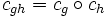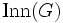# Questions:Inner automorphism

Jump to: navigation, search

This is a list of common questions about inner automorphisms. See also inner automorphism (the main page on the subject).

Q: Why is an inner automorphism an automorphism? How is the group structure of a group related to that of its automorphism group?

A: This basically is a straightforward verification that involves some symbol manipulation. See group acts as automorphisms by conjugation, which not only shows that conjugations by elements are automorphisms, but also that the conjugation by$gh$ is the composite of conjugations by$g$ and$h$. In other words, if$c_g$ denotes conjugation by$g$, we have$c_{gh} = c_g \circ c_h$ for all$g,h \in G$.

We thus obtain a homomorphism of groups from$G$ to$\operatorname{Aut}(G)$ (the automorphism group of$G$) sending each$g \in G$ to the conjugation map$c_g$. The kernel of this is the center$Z(G)$ and the image is the inner automorphism group$\operatorname{Inn}(G)$. We thus have, from the first isomorphism theorem, that$\operatorname{Inn}(G) \cong G/Z(G)$.

Q: Given an inner automorphism, is there a unique element for which this is the conjugation operation? If the element is not unique, is there some natural way of picking an element?

A: The conjugating element need not be unique. In fact, the set of candidates for conjugating element for a given inner automorphism is a coset of the center. This is because the center is the kernel of the homomorphism$G \to \operatorname{Aut}(G)$ given by conjugation action. The conjugating element is unique only in the case of a centerless group.

Moreover, there is no natural choice of conjugating element in general. In fact, even making a uniform choice may require use of the axiom of choice, since we need to choose infinitely many coset representatives.

Further, it is not necessary that we can make the choice in such a way that the product of two of the chosen representatives for the coset of center is again a chosen representative. In other words, we may not be able to choose our representatives so as to form a group. We can make such a choice only in a group whose center is a direct factor.

Q: It is clear that an inner automorphism can be extended to any bigger group as an inner automorphism of that group -- simply use the same conjugating element. Are there other automorphisms that can always be extended?

A: No. This is hard to prove. The direction you mentioned is that inner implies extensible. The reverse direction -- that any extensible automorphism is inner -- is nontrivial. Further information: extensible equals inner (specific proof), extensible automorphisms problem (more discussion)

Q: It is clear that an inner automorphism can be pulled back via any quotient map, i.e., an inner automorphism of a quotient arises from an inner automorphism of the original group. Are there outer automorphisms for which this is always true?

A: No. This is similar to the previous question, and is proved using similar methods. The direction you mention above is inner implies quotient-pullbackable. The other, harder, direction is quotient-pullbackable equals inner. For more discussion, see extensible automorphisms problem.

Q: An inner automorphism sends every element to within its conjugacy class. Are there outer automorphisms that do so?

A: Yes. An automorphism that sends every element to within its conjugacy class is a class-preserving automorphism. See inner implies class-preserving, class-preserving not implies inner, and group in which every class-preserving automorphism is inner.